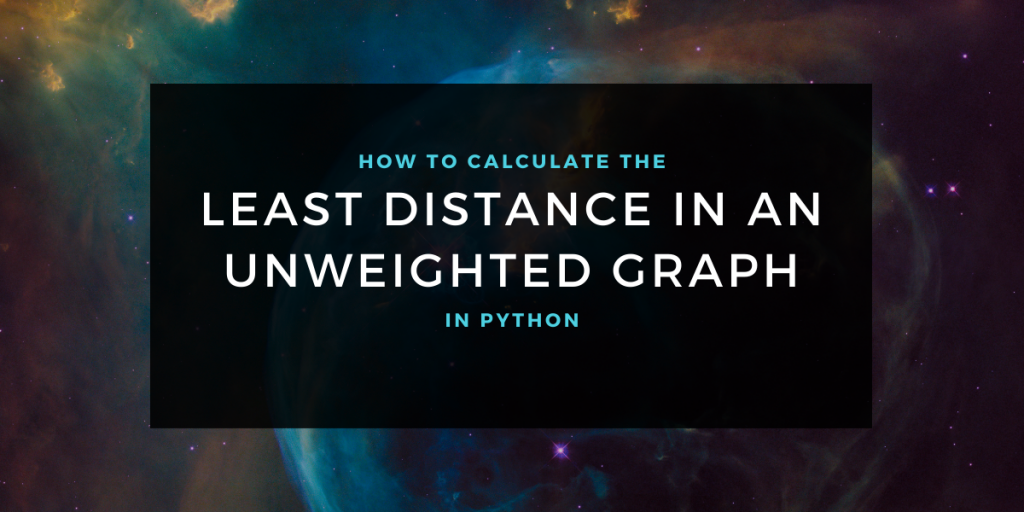# Calculating the Distance Between Nodes in an Unweighted GraphGraph traversal algorithms have various applications. One of the applications is to find the least distance between two nodes of a graph. In this article, we will implement an algorithm to find the least distance in an unweighted, fully connected graph in python using breadth-first graph traversal algorithm.

## Using The BFS Algorithm for a GRaph

Breadth-first search is a graph traversal algorithm in which we traverse each vertex of the graph exactly once by starting from any single vertex. For each selected vertex we first print the vertex and then we print all of its neighbors. This process is continued till all the vertices are traversed. While traversing a graph with breadth-first search, it looks like we are moving in layers starting from the selected vertex.

The implementation of the BFS algorithm for a graph is as follows. In this algorithm, we have assumed that the graph is unweighted, undirected, and is fully connected.

```def bfs(graph, source):
Q = Queue()
visited_vertices = set()
Q.put(source)
visited_vertices.update({0})
while not Q.empty():
vertex = Q.get()
print(vertex, end="-->")
for u in graph[vertex]:
if u not in visited_vertices:
Q.put(u)
visited_vertices.update({u})
```

## Determining the Least Distance Between Two Nodes of an Unweighted Graph

We can use the breadth-first search algorithm to find the least distance to all nodes from a source by making certain modifications to the algorithm.

Given the source and the adjacency list representation of the graph, we will declare a list that will contain all the visited vertices and we will also create a dictionary in which keys of the dictionary determine the vertex and values determine the distance between the current vertex and source.

The modification to the BFS algorithm here will be that whenever we process a vertex v, we will update the distance of its neighbors. The distance of the neighbors of v from the source will be equal to the distance of v from the source added one.

## Algorithm for determining the least distance

As we have a general idea of how to determine the least distance from the source to each vertex, we will formulate the algorithm for the same.

```Algorithm Least Distance:
Input: Graph(Adjacency list) and Source vertex
Output: A list with distance of each vertex from source
Start:
1.Create an empty queue Q.
2.Create an empty set to keep record of visited vertices.
3. Create a dictionary in which keys of the dictionary determine the vertex and values determine the distance between current vertex and source.
4.Insert source vertex into the Q and Mark the source as visited.
5.If Q is empty, return. Else goto 6.
6.Take out a vertex v from Q.
7.Insert all the vertices in the adjacency list of v which are not in the visited list into Q and mark them visited after updating their distance from source.
8.Goto 5.
Stop.
```

## Implementing the Graph Traversal to Calculate the Least Distance

As we have formulated the algorithm for determining the least distance of vertices from a source, we will implement the algorithm and execute the algorithm for the graph given in the following image.

The implementation of the algorithm in python is as follows.

```from queue import Queue

myGraph = {0: [1, 3], 1: [0, 2, 3], 2: [4, 1, 5], 3: [4, 0, 1], 4: [2, 3, 5], 5: [4, 2]}

def leastDistance(graph, source):
Q = Queue()
# create a dictionary with large distance(infinity) of each vertex from source
distance = {k: 9999999 for k in myGraph.keys()}
visited_vertices = set()
Q.put(source)
visited_vertices.update({0})
while not Q.empty():
vertex = Q.get()
if vertex == source:
distance[vertex] = 0
for u in graph[vertex]:
if u not in visited_vertices:
# update the distance
if distance[u] > distance[vertex] + 1:
distance[u] = distance[vertex] + 1
Q.put(u)
visited_vertices.update({u})
return distance

print("Least distance of vertices from vertex 0 is:")
print(leastDistance(myGraph, 0))

```

Output:

```Least distance of vertices from vertex 0 is:
{0: 0, 1: 1, 2: 2, 3: 1, 4: 2, 5: 3}
```

## Conclusion

In this article, we have implemented an algorithm to find the least distance between a source and other vertices of a graph using a depth-first search traversal algorithm. Stay tuned for more informative articles.